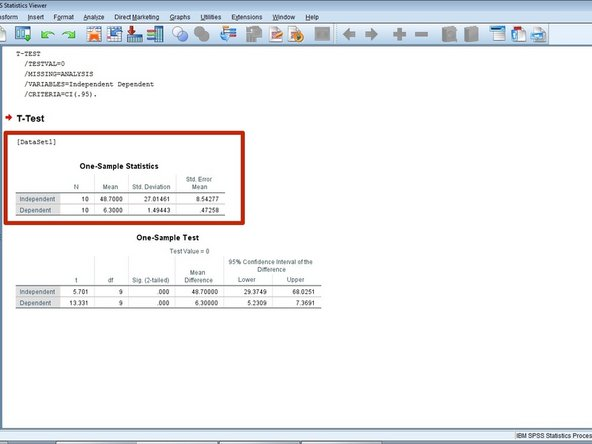Zum Hauptinhalt wechseln

Englisch
Deutsch

## Schritt 12 übersetzen

Schritt 12Understanding the Results
• The first chart is the Descriptive Statistics:

• The first column is the number of participants.

• The second column is the mean.

• Mean: the average of a set of values.

• The third column is the Standard Deviation.

• Standard Deviation: a measure of the expected deviation of the results.

• The fourth column is the Standard Error of the mean.

• Standard Error: the distribution of sample mean around the population mean.

Hier Übersetzung einfügen

Hier Übersetzung einfügen

Hier Übersetzung einfügen

Hier Übersetzung einfügen

Hier Übersetzung einfügen

Hier Übersetzung einfügen

Hier Übersetzung einfügen

Hier Übersetzung einfügen

Deine Beiträge sind lizenziert unter der Open-Source Creative Commons Lizenz.